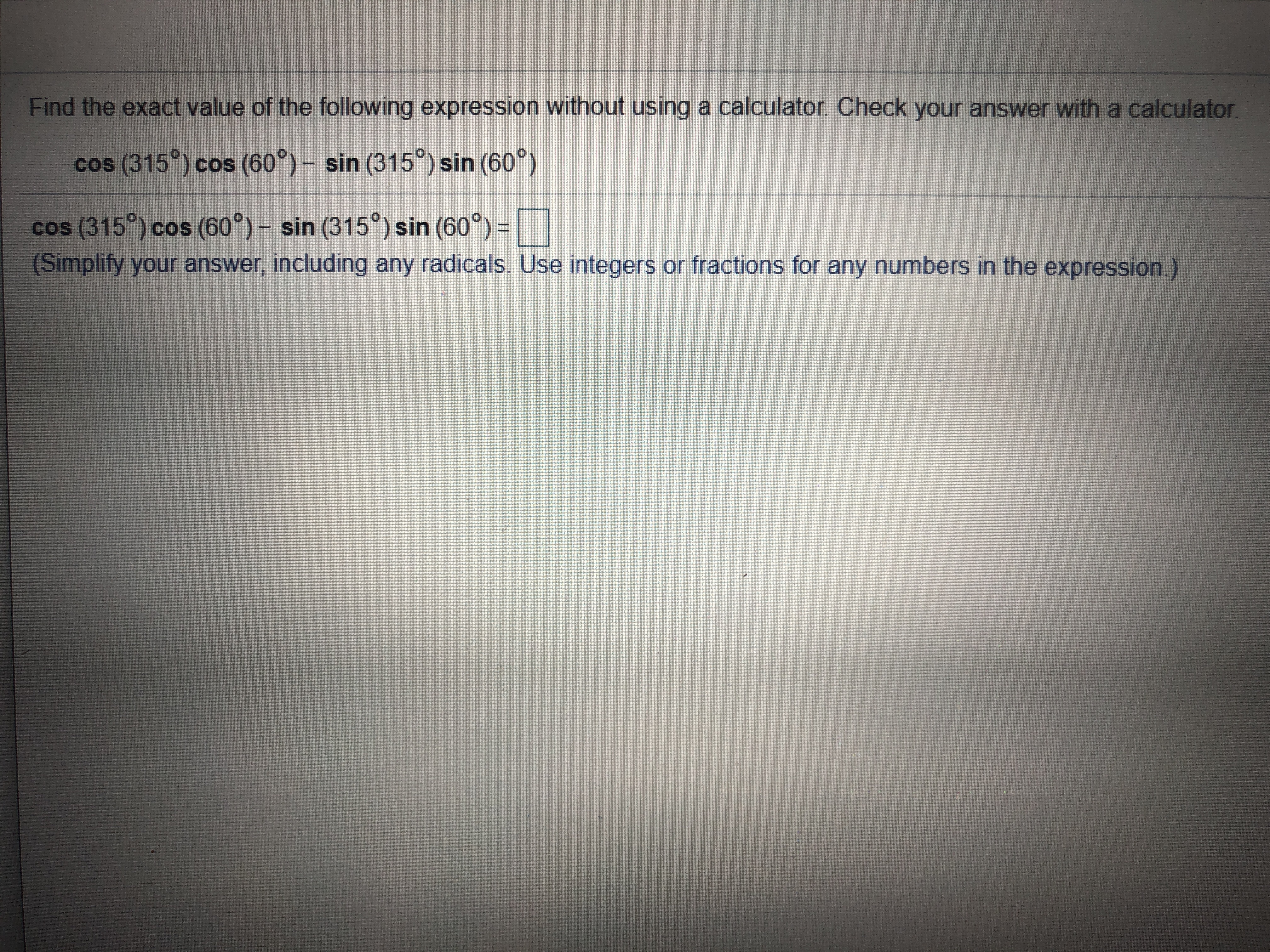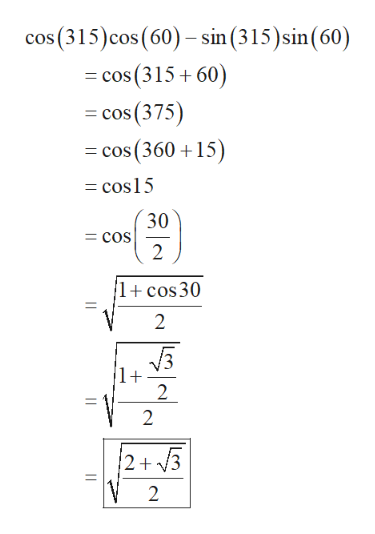Find the exact value of the following expression without using a calculator. Check your answer with a calculator.cos (315°) cos (60°)- sin (315) sin (60°cos (315) cos (60°)- sin (315) sin (60)(Simplify your answer, including any radicals Use integers or fractions for any numbers in the expression.)C

Question

Can you explain how to answer this question? HOW do I arrive at an answer without using a calculator?help_outlineImage TranscriptioncloseFind the exact value of the following expression without using a calculator. Check your answer with a calculator. cos (315°) cos (60°)- sin (315) sin (60° cos (315) cos (60°)- sin (315) sin (60) (Simplify your answer, including any radicals Use integers or fractions for any numbers in the expression.) C fullscreen
Step 1

Obtain the exact values of the given expression as follows. Recall the given facts.

Step 2

Obtain the exact valu...help_outlineImage Transcriptionclosecos (315) cos (60)-sin (315)sin (60) cos (315 60) =cos (375) = cos (360 15) cos15 30 = cOs 2 1+cos30 2 2 2 2+ 3 2 fullscreen

Want to see the full answer?

See Solution

Want to see this answer and more?

Our solutions are written by experts, many with advanced degrees, and available 24/7

See Solution
Tagged in

Other